Flow Measurement

# Flow Measurement - Notes | Study Mechanical Engineering SSC JE (Technical) - Mechanical Engineering

 1 Crore+ students have signed up on EduRev. Have you?

FLOW MEASUREMENT

 Device Measurement Venturimeter rate of flow (discharge) Flow nozzle rate of flow Orifice meter rate of flow Bend meter rate of flow Rotameter rate of flow Pitot tube velocity Hot wire anemometer air & gas velocity Current meter velocity in open channels

Venturimeter 

• Cone angle of convergence side (20°) is greater than cone angle of divergence side (5° to 7°). 
• Position of venturimeter does not play any role in discharge thus it is constant for horizontal, inclined or vertical position.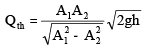Qac = Cd Qth
Cd = coefficient of discharge = 0.95 to 0.98

• Throat diameter is known as size of the venturimenter.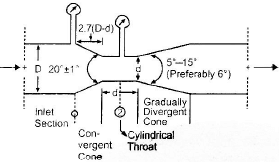Orifice meter 

• It is a cheaper arrangement but has more energy loss.

Cd for orifice meter = 0.68 - 0.74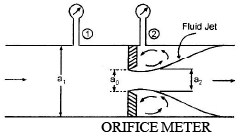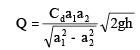Cd for orifice meter = 0.68 - 0.74

a1 = πd2/4

a2 = πdc2/4

Pitot Tube 

• It is based on principle of conversion of kinetic head into pressure head. The point at which velocity reduces zero is called stagnation point.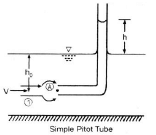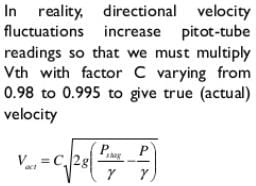• For theoretical velocity, we do not multiply the equation by C.
• Velocity head is indicated by the difference in liquid level between the Pitot tube and the piezometer. The Pitot tube measures the total head and therefore known as total head tube.

Hydraulic Coefficients

• Contraction coefficient (Cc) =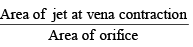• Coefficient of velocity of (Cv) =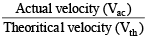• Coefficient of discharge (Cd) =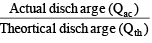• Cd = Cv.C

Flow through orifice in the tank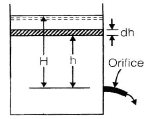Time required to lower the level from H to h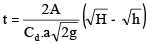Discharge through orifice when head over orifice is h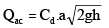• Rectangular Weir/Notch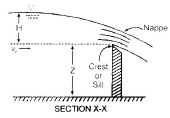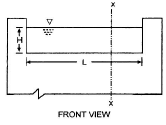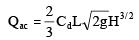L = length of crest H = head above crest 

• Triangular weir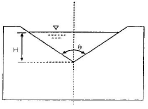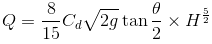• Trapezoidal weir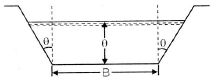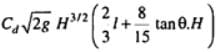The document Flow Measurement - Notes | Study Mechanical Engineering SSC JE (Technical) - Mechanical Engineering is a part of the Mechanical Engineering Course Mechanical Engineering SSC JE (Technical).
All you need of Mechanical Engineering at this link: Mechanical Engineering

## Mechanical Engineering SSC JE (Technical)

6 videos|97 docs|55 tests

## Mechanical Engineering SSC JE (Technical)

6 videos|97 docs|55 tests

Track your progress, build streaks, highlight & save important lessons and more!

,

,

,

,

,

,

,

,

,

,

,

,

,

,

,

,

,

,

,

,

,

;